﻿ Yield Curve Estimation: An Empirical Evidence from the Tunisian Bond MarketPublications are Open
Access in this journal
Article Versions
Export Article
• Normal Style
• MLA Style
• APA Style
• Chicago Style
Research Article
Open Access Peer-reviewed

### Yield Curve Estimation: An Empirical Evidence from the Tunisian Bond Market

Aziz Chouikh , Rania Yousfi, Chehir Chehibi
Journal of Finance and Economics. 2017, 5(6), 300-309. DOI: 10.12691/jfe-5-6-6
Published online: December 13, 2017

### Abstract

Our paper aims to model the yield curve that corresponds to a graphical representation of the yields offered by the bonds of the same issuer according to their maturity, from the shortest to the longest expiration date in the Tunisian bond market (TBM). To get to our objective, we will compare the Nelson-Siegel modeling strategy, which is most often used for the analysis and the hedging of the interest rate risk of portfolios with known flows in practice, to the Svensson modeling strategy, which is the extension of the Nelson-Siegel model. Our sampling data statistically support the evidence that the more appropriate yield curve for the TBM is that estimated by the Nelson-Siegel model.

### 1. Introduction

Our paper aims to better understand the behavior of the yield curve in order to be able to create a more efficient and reliable one. Our yield curve will be essentially based on the Nelson Siegel and the Svensson models since those are widely used amongst the financial institutions and adapted to less liquid and less developed markets similar to the Tunisian bond market (TBM).

The Nelson-Siegel and Svensson models are zero-coupon parametric models with the advantage of taking into account the different deformations of the yield curves, to allow a dynamic analysis of the market with time-varying parameters that are estimated from market data, and represent the curve by a smooth surface.

With the aim of facilitating for investors the access and consultation of all monetary-rate and bond-rate curves currently broadcast in Tunisia, Tunisie Clearing (TC){1} has launched an information centralization initiative on its website. In March 2016, TC started publishing a yield curve for treasury bills inspired by the practices of different markets around the world.

While working with the available information on TC website, a gap has appeared. The data used to make the yield curve reflect the whole market for intra primary dealer operations and all the operations that are out of the market which leads us to think that TC yield curves may be biased, so it is mandatory to point out a yield curve which is more reliable and efficient.

To do so, we will estimate the yield curve via Nelson-Siegel and Svensson models. Then we will compare them to TC’s yield curve, and we will choose the best one as a benchmark for the TBM.

The remainder of our paper is organized as follows. Section 2 deals with the literature review. Section 3 points out the yield curve modeling framework. Section 4 copes with the empirical methodology. In section 5, we run the yield curve estimations. Section 6 exhibits results and findings. Finally, in section 7, we conclude.

### 2. Literature Review

The term interest rate structure (or yield curve) is the function that, combines on a given date, the level of the associated interest rate for each maturity. According to Gbongue & Frederic Planchet 1, there are two families of yield curves, market curves and implied curves:

Market curves are constructed directly from the market quotations. It is about the swap curve and yield curve of government bonds. Implied curves are constructed indirectly from the market quotations for instruments such as bonds and swaps (zero-coupon (ZC) yield curve, forward yield curve, instant forward yield curve and finally yield curve at par).

The literature provides two methods to construct a ZC curve; the bond pricing approaches and approaches to yields. An abundant literature exists on the methods of construction of the yield curve. They can be grouped into two main groups: those using parametric methods and those based on non-parametric techniques. Other researchers have proposed to group them into three categories: First, based on the spline functions. Second, those that a priori postulate a function class and finally, non-parametric techniques (Roncalli 2). In this paper, we propose to group the methods of construction of the yield curve into 4 categories: methods using regression models, methods derived from empirical models, methods using parametric models, and finally methods using bootstrapping method.

The traditional method of constructing a yield curve is to represent the rate of return of a series of bonds by maturity. Regression models respond to this method. In these models, bond yields are a function of several explanatory variables (maturities, coupon rates). The parameters are estimated by minimizing the squared error between theoretical yield derived from the model and the bond yield observed in the bond markets. In this category, it is important to mention the the work of Mcenally 3, Dobbie and Wilkie 4, Dobbie and Wilkie 5, Bolder and Streliski 6.

The second category is composed of empirical models. The general idea is to adjust the discount factor by an appropriate mathematical function and then extract the parameters. These are obtained by minimizing the squared deviation between the theoretical price derived from the model and the price of the bonds observed on the bond markets. Several mathematical forms exist in the literature to adjust the discount factor. First, we have the "spline" function, which includes the quadratic spline{2}, the spline cubic, the exponential spline, B-splines{3} (see Mcculloch and Kochin 7).

In fact, the weakness of the Lagrange interpolation is that the interpolation error increases with the sample size N{4}. This is expressed experimentally by large oscillations of the interpolation polynomial, even if the function is very simple. For example, when interpolating the function x →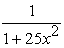in points uniformly distributed over the interval [-1, 1], the Lagrange polynomials{5}does not converge towards F (the function F). Hence the idea of interpolating by polynomial functions by bits, of which the degree does not increase with the number of interpolation points. For example, the function X1 which is affine on each interval [,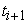] and interpolates a function F class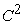satisfied, according to the theorem{6} applied on each interval:As one wishes to interpolate by functions more differentiable than X1, we look for the interpolant in the space of the polynomial classfunctions, of degree k on each interval [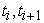] called spline functions (the terminology was introduced by Schoenberg in 1946) (Pansu 8).

These methods have been criticized for having undesirable economic properties and are often perceived as "black boxes". Carriere 9 pointed out that the survival models used by actuaries to explain losses can be used as price models, which implies that the function can be approximated by a survival function{7}.

The third category is composed of parametric models. The most used parametric model is the Nelson and Siegel 10 model. Since its appearance, it has been adopted by many experts from the professional world. It is used by many central banks, policy makers and fixed-income portfolio managers. Also, the Nelson-Siegel model has known a great success in academic research. Indeed, Dullman, Klaus, Marliese Uhrig-Homburg, and Windfuhr 11 used the Nelson-Siegel model to construct a ZC rates adapted to the German bond market.

The Nelson-Siegel model has the advantage of being parsimonious and its parameters have an economic significance. However, it still presents some disadvantages. Indeed, it does allow to reconstitute all forms of yield curves that can be found on the market. It lacks the flexibility of adjustment for maturities over 7 years. The Nelson-Siegel model class is in practice most often used for the analysis and the hedging of the interest rate risk of portfolios with known flows. The Svensson model is the extension of the Nelson-Siegel model to allow an additional curvature (Pierre-E.Therond 12).

The work of Roncalli 2 showed the existence of two classes of models rates for valuation of financial assets: models of no arbitrage opportunity (NAO) and general equilibrium models.

The basic assumption adopted in the NAO models is that in a sufficiently fluid market{8} there is no opportunity for arbitrage that means it is not possible to make profits without taking a risk and this is called a no-arbitrage opportunity model (absence of arbitrage opportunities (AAO)), which is a basic principle in derivatives pricing). At the level of the no-arbitrage opportunity models (NAO or AAO), we can cite the work of Vasicek 13, Brennan and Schwartz 14, Ho and Lee 15, Heath, Jarrow and Merton 16. In the other hand, the theory of general equilibrium studies the allocation of resources in the context of a market economy with perfect competition. It highlights how markets and prices coordinate economic activities. The general equilibrium models propose theories about the nature of the stochastic process that interest rates follow. Unlike the NAO models, these models are based on expectations of future interest rate movements, but not on the yield curve as observed at the initial date (Gbongue & Frederic Planchet 1).

The fourth category is composed of the bootstrapping models. The bootstrapping method is a procedure for reconstructing a ZC curve step-by-step, that means maturity segment by maturity segment. Having a single unknown and a single equation (market price = theoretical price) we can determine the ZC rate. The price of the second bond take an equation with two unknowns (the first coupon to be discounted at the first year's rate and the second coupon with the second year's rate), The rate for the first year being determined through the first bond, we can find without worry the rate of the second year, and then we can find all the ZC rates. The big problem with this method is that we have to have the bonds with successive maturities to be able to do the bootstrapping, something that is not always evident in the emerging markets (Annaert, Anouk G.P.Glaes, Mark J.K.De Ceuster, & Hairui Zhang 17).

More recently, we observe models for the construction of yield curves that takes into account its dynamic character. We can enumerate first the principal component analysis models (PCA); when several (more than two) quantitative variables are studied simultaneously. The difficulty comes from the fact that the individuals studied are no longer represented in a plan, space of dimension 2, but in a space of larger dimension (e.g. dimension 4). The objective of the Principal Component Analysis (PCA) is to return to a space of reduced size (for example 2) by deforming the least possible reality. It is therefore to obtain the most relevant summary of the initial data. Mathematically, PCA is a simple base change: switch from a representation in the canonical basis of initial variables to a representation in the basis of the factors defined by the vectors specific to the matrix of correlations.

The Nelson-Siegel dynamic, and the Functional Signal plus Noise (FSN) are proposed to analyze the dynamics of a wide range of yields or asset prices in which observations are contemporary functionally related. FSN models are used to predict yield curves of the Treasury in the next month “m+1”; m being the current month (Clive G. Bowsher and Roland Meeks 18). Among the authors who used the PCA as a technique for estimating the yield curve zero, we can cite the work of Litterman and Scheinkman 35. Other authors apply the PCA method to construct the term structure of interest rates in a Heath-Jarrow-Morton (HJM) model. HJM model is an arbitrage model and unlike equilibrium models, it tries to automatically adjust to the structure of interest rates (Racicot & Theoret 20).

In the same context, Leoni 21 discusses in detail the applications of the PCA in the determination of interest rates. Wilmott 22 discusses the PCA method while considering the estimation of volatilities in HJM models. Diebold and Li 23 introduce a dynamic version of the model of Nelson Siegel, while Bowsher and Meeks 18 applies the "Functional Signal plus Noise (FSN) "to model and predict the zero-rate curve from treasury.

Other interesting methods of estimating the yield curve are worth noting. Such as Filtering and Garch{9} techniques. Pennacchi 24, Babbs and Nowman 25, and Babbs and Nowman 26 use the Kalman filter to estimate gaussian affine models. Lund 27 uses a non-linear filter to estimate an affine model from bonds with coupons. Finally, Brenner et al. 28 models the short-term rate as a GARCH stochastic process (Gbongue & Planchet 1).

### 3. The Yield Curve Modeling Framework

3.1. The Nelson-Siegel Model (1987)

The Nelson-Siegel model class is in practice most often used for the analysis and the hedging of the interest rate risk of portfolios with known flows.

In fact the Nelson-Siegel model is distinguished by its dynamic analysis with parameters that change over time and by its simple analysis with few parameters to estimate, consistent with illiquid and less developed markets. It is used for the construction of a smooth surface curve and also for the modeling of different deformations of the curve (level, slope, curvature). The underlying model is expressed by the following equation (1):(1)

Where

R: ZC yield-to-maturity rate,: level factor; it is about the long rate,: Slope factor; it is the difference between the short rate and the long rate,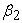: Bending factor (convexity) and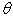: The remaining period divided by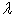.

The Nelson-Siegel model has some inconvenient which consist of; first, the lack of flexibility of adjustment for the more than 7 years maturities and the absence of modeling curves at a hump and a trough.

3.2. The Svensson Model (1994)

Svensson 19 presented an extension of the functional model of Nelson-Siegel.

Svensson findings allowed overcome the disadvantages of the basic model.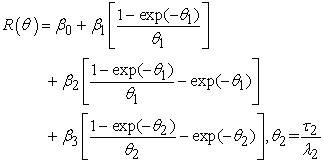(2)

Where

R: ZC yield-to-maturity rate,: level factor; it is about the long rate,: Slope factor; it is the difference between the short rate and the long rate,: Bending factor (convexity),: The second hump term,: The remaining period divided by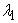and: The remaining period divided by.

### 4. The Empirical Methodology

4.1. Data
4.1.1. The Choice of Lines

The sample will be composed of Treasury bills which are; long-term treasury bonds (newly issued during the current year), short-term treasury bonds (newly issued) and finally, ZC treasury bonds (without restrictions). The national loan is excluded because it is a punctual and particular issue.

4.1.2. The Choice of the Underlying Market

In the Tunisian context, the primary market is more active than the secondary market so here we choose to work on a history over 1 month for this market and a history over 2 months for the secondary market to reach more equilibrium between the markets in our sample. Thus, the primary market will not overwhelm the secondary market.)

To collect relevant data from the primary market we apply the validity the time test1{10} in order to limit the sample in an interval of historic over one month. The time test bases on the difference between the date of today-1 and the operation date{11}, we post “True” if the Treasury bill has an historic of one month or less and “False” if the historic exceed one month.

Then we created a button that we named “Export” using the excel macro in order to extract the final and clean primary market sample. The “Export” button will transfer the treasury bills that have a time test with the result “True” to another clean excel sheet named “Sample-p”.

Relatively, the secondary Market is an inactive market, in spite of the conditions imposed by the specifications. So here we choose to take a history over 2 months in order to make the secondary market more effective in our sample and to reach more equilibrium between the two markets.

We applied two tests on the data of the secondary market. The first test was the time test 2{12} which is based on the difference between the today’s-1 date and the operation date. The second test is applied only on the intra primary dealer treasury bills, it measures the spread between the weighted average rate (WAR) and the rates of the FMC{13} (Financial Market Council), and this spread must be lower than 15 basis points{14}. If the Treasury bill respond to these conditions, excel will post “True” otherwise it will post “False” as a result.

Just like the work done on the primary market data an “Export” button was created in the secondary market sheet in order to transfer all the treasury bills with the “True” result to another clean sheet that we named “Sample-s”.

Finally, the combination of the two markets, primary market and secondary market in another work sheet named “Sample” will enable us to have the relevant database.

4.2. The Methodology
4.2.1. The Average of the Weighted Average Rate

In order to find the average of the weighted average rates

(WAR), we need to go over three steps:

Step1: Starts with the calculation of the product of the quantity and the weighted average rate (Quantity*WAR). Then we sort the sample by ISIN code, this allows calculating the average of the quantity and the (Quantity1{15}*WAR) of all the treasury bills with the same ISIN code. Finally, to find the average WAR{16} we divide the (quantity1*WAR) by the quantity2{17}.

Step2: The objective here is to find the theoretical prices. We create first a new sheet named “Price-yield” that imports the yields from the Average WAR of the “Sample” sheet then for every long-term Treasury bill we create a new independent sheet with the name of each treasury bill. The short-term and ZC treasury bills were summarized into one sheet. In each Spreadsheet we are looking for the theoretical prices and to do so we need to import yields from the “Price-yield” sheet to enable Excel to apply the available equation to find the price. Once the theoretical price is found it is exported into the “Price-yield” spreadsheet.

Step 3: Now we want to estimate our yield curve, so we need first to create a new spreadsheet that contains the equations of ZC rates, re-evaluated prices, import the target prices from the “Price-yield” sheet and also we need to put all the variables which are the betas;and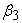(for the Svensson model) and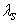;and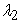(for the Svensson model). As a start we can work with the variables available on Tunisie Clearing website to calculate our ZC rates and, as a result, the prices. Once the prices are found, we optimize the squared difference between the target prices and the re-evaluated prices by using Excel solver. Thus, we find the optimal variables (and). Then, the ZC rates are determined and the corresponding yield curve is created.{18}

4.2.2. Pricing

With the aim to re-evaluate the Bank’s Treasury bills portfolio we created a new sheet named “REEVAL”. In this sheet we import the bid and ask prices calculated in each Treasury bill sheet.

Step 1: we calculate the bid and ask prices using the facial rate, import the target prices from the curve spreadsheet, and add the spread to each price. The spread is fixed at 10 basis points if the Treasury bill is acquired before 2020, and 5 basis points if the Treasury bill is acquired after{19}.

Step 2: we use Excel solver to find the bid and ask yields that enable us to make bid and ask prices equal to the target prices. Once all the prices and yields for the bid and ask are found, we import them into the “REEVAL” spreadsheet.

Step 3: Finally, to more automate the work we created a new sheet named “Tanit” in which the solver work in all the treasury bills sheets will be automatic thanks to excel macro.

The sample is daily updated based on the database available on TC website. We add all the newly issued treasury bills of every day to the sample of the primary market and secondary market, and launch the tests in order to get the clean and final updated sample. We calculate the average WAR. Thus, the yield curve will be automatically prepared. For the pricing, we just need to run the Excel solver in the “Tanit” spreadsheet for all the treasury bills. Thus, the bid and ask yields and prices will be automatically updated.

The final step is the comparison of the results evidenced by different models (Nelson-Siegel and its extension Svensson).

### 5. Yield Curve Estimation

The yield curve corresponds to a graphical representation of the yields offered by the bonds of the same issuer according to their maturity, from the shortest to the longest. The most common yield curve, which serves as a reference for the entire bond market of a given country, is that of government bonds.

The shape of the yield curve on sovereign bonds provides information on investors' expectations about the risk of default of the issuing country and the level of inflation and future interest rates. As such, it is a good indicator of the economic and financial health of the issuing country. Quantitative easing policies of central banks can also strongly influence it.

5.1. The Shape of the Yield Curve is an Indicator of Economic and Financial Health

In a stable economic environment{20} marked by low inflation and sustainable public debt, bond yields increase with the maturity of the securities, that means that interest rates rise steadily as maturity of the security is removed.

• Figure 1. Normal Yield curve
• Figure 2. Inversion Yield curve
• Figure 3. Steepening yield curve
• Figure 4. Flatting Yield Curve

This is because the longer the maturity date, the greater the risk of occurrence of events that could adversely affect the value of the bond. Indeed, the further away you go, the greater the uncertainty about the repayment capacity of the issuer or the level of interest rates or inflation. Under these conditions, investors require a risk premium to lend over long maturities compared to lending on short maturities.

However, since government bonds are generally considered to be "safe" securities, the risk premiums attached to the removal of maturities are fairly small. On the longest maturities, at 20, 30 or 50 years, they can even be virtually zero because over these very long-term horizons, default risk as well as interest rate and inflation risks can be considered as overall identical. This is why the yield curve on government bonds of the main developed countries generally has an increasingly concave form, as shown in the figure.

5.2. The Risk-Based Yield Curve
• Figure 5. risk-based yield curve

Given investor risk aversion{21}, the demand for the least risky securities is higher than other riskier securities. As a result, interest rates on the entire maturity of an issuer are lower the lower the risk perceived by investors for these securities.

Thus, generally, bonds issued by companies are considered riskier than those issued by governments. This is why there is a risk premium buried in bond prices in relation to sovereign bonds. This risk premium is reflected in higher interest rates for each maturity date, which translates into a yield curve similar to that of government bonds but shifted upwards.

Similarly, the risk perceived by investors on the bonds of the different issuing countries varies according to the quality of their signature. For example, a country with the best rating would be able to borrow on the bond market at lower interest rates than a lower rating country. This difference in risk between the two countries will also be reflected in a downward-shifted yield curve for the issuer with the best rating.

However, the shape of the yield curve is not fixed. It may change significantly as a result of a marked change in the monetary or budgetary situation of the issuing country.

5.3. Yield Curve's Shapes Interpretation
• Figure 6. The steepening of the yield curve

The steepening of the yield curve corresponds to a situation in which the shape of the bond interest rate curve changes from a concave curve to a shape closer to a straight line, Long-term interest rates.

The steepening of the yield curve occurs when investors demand high risk premiums to lend on long-term maturities to compensate for an inflationary risk or future budgetary slippage which they judge to be higher than the current level. The steepening of the yield curve is therefore associated with expectations of a rise in forward inflation or a deterioration in the fiscal position.

The inversion of the yield curve occurs when short-term rates become higher than long-term rates. This is typically what happens in a situation of strong inflation, which leads the central bank to raise its short-term interest rates, which increase over all short-term maturities (from three months to two years). If the central bank is credible in the eyes of investors, then they anticipate a fall in future inflation and reduce the risk premium attached to long-term bonds.

• Figure 7. Inversion yield curve

An inversion of the yield curve is often associated with periods of economic overheating, with strong activity causing inflationary pressures. The latter push the central bank to intervene via the rise in short-term interest rates that eventually lead to an economic slowdown. The inversion of the interest rate curve is thus generally considered as an indication of the onset of a recession in the medium term.

• Figure 8. Flatting yield curve

The flattening of the interest rate curve corresponds to a situation in which long-term rates decline and approach the level of short-term interest rates while remaining higher.

This situation can result from different causes. This may include changes in investor expectations as to the level of long-term interest rates. If, for example, they believe inflation will decline in future years, the premium required to buy long-term bonds will decline.

Yet other factors may also result in a flattening of the interest rate curve. In particular, if the fiscal position of a highly indebted state improves, this country's medium- and long-term default probability is reduced, which will result in a lower risk premium required by investors to subscribe issuance of securities on the longest maturities.

Moreover, the combination of a low inflationary environment and an abundance of liquidity at the global level favors the search by international investors for investments that are both risky and offer higher returns than those offered on short-term securities term. Demand for long-term bonds from the main developed countries is increasing, pushing long-term rates down.

### 6. Results and Findings

6.1. Nelson-Siegel and Svensson Models
• Figure 9. Nelson-Siegel yield curve

The Nelson-Siegel model is most often used for the analysis and the hedging of the interest rate risk of portfolios with known flows in practice.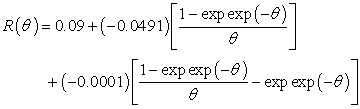(3)

WithWhere

R: ZC yield-to-maturity rate,: level factor; it is about the long rate,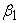: Slope factor; it is the difference between the short rate and the long rate,: Bending factor (convexity) and: The remaining period divided by.

• Figure 10. Svensson yield curve

The Svensson model is the extension of the Nelson-Siegel model to allow an additional curvature.(4)

With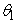=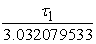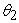=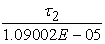Where

R: ZC yield-to-maturity rate,: level factor; it is about the long rate,: Slope factor; it is the difference between the short rate and the long rate,: Bending factor (convexity),: The second hump term,: The remaining period divided byand: The remaining period divided by.

Using the Nelson-Siegel and Svensson models, a normal yield curve was pointed out, reflecting the statistical evidence that the higher the maturity is, the higher the interest rate is (i.e. The first derivative of the yield to maturity with respect to the maturity is positive).

The main reason behind the upward-sloping yield curve in the TBM could be related to the lack of market liquidity and visibility of the future economic situation in the banking sector and the Tunisian economy as a whole.

As it is known lately, the Tunisian government has been raising its level of nominal debts, which is divided into two different types; internal debts and external debts. The huge problem for our government is the increasing external debt, which is supported by the internal debt. To solve the equilibrium problem between the two main sorts of debts, the Tunisian government needs to be financed by the banking sector, and this funding will be mainly set up through the primary bond market by the adjudication of new treasury bills (T-bills). Nowadays, banks have started to accept the participation in those adjudications with the condition of the higher interest rates, so longer term treasury bonds since this kind of bonds has a higher revenue given a higher level of risk (in the case of the government bonds we cannot talk about different ratings since all the bonds are rated as the less risky bonds, so here we relate the risk with the maturity of the bond. That means if we have two different bonds A and B with different maturities. We have also the information that the maturity of A is higher than the maturity of B so here we can say that the bond A is more risky than the bond B since we don’t have a clear vision of the future situation of the economy). This condition has caused the ascendant normal shape of the yield curve.

6.2. Nelson-Siegel-Svensson and TC Yield Curves
6.2.1. Short-term Horizon

The interest rate quotes are usually based on the supply and demand of money on the money market. In Tunisia, we can benchmark our quotations on 3 possible rates:

• Figure 11. Nelson-Siegel, Svensson and Tunisie clearing yield curves

6.2.1.1. Placement Rate

We have two types of interest rates: first, the certificate of deposit which are debt securities issued by the credit institutions (banks and leasing organizations) where the duration of which shall be, at least, ten days and not more than five years. These debt securities are subscribed by companies and physical persons. Such certificates may not be prepaid or have a premium of refund. Also, they have a fixed deadline too.

The second type is composed of the delivered pension which is also referred to as a bond repurchase agreement. A pension is a contract whereby an institutional investor or a company can exchange its cash for a definite period against financial securities. The problem here is that this kind of rates has a high volatility (for example, rates of the certificate of deposit varies from a bank to another), so it will not be possible to base our quotations on this kind of rates and this problem makes us think about the two other types.

6.2.1.2. TUNIBOR (Tunis Inter-Bank Offered Rate)

TUNIBOR is also called the benchmark interest rate on the Tunisian dinar. It is an indicative average interest rate at which active banks in the dinar money market are willing to lend unencumbered liquid assets for eight different maturities ranging from a day to twelve months: one day, one week, one month, two months, three months, six months, nine months and twelve months. The TUNIBOR is still in the test phase since this rate has a low volume. So, currently it is only used for information.

6.2.1.3. Tender Rate

The rate of the tender is the money market floor rate. Since 1988, the Central bank of Tunisia (CBT) has applied the single interest rate method. Since 2004, the CBT has adopted the mixed method based on the notion of price rather than on the notion of counterparties.

As a matter of fact, it is not possible in the Tunisian context to base the quotation neither on the placement rates nor on the TUNIBOR as we said above. We are obliged to let the tender rate, which is in the neighborhood of 4.25%, be the base of our quotation during the related week (from 04/10/17 to 04/14/17).

As we can see in the figure 12 above, the Nelson-Siegel curve and the TC curve are actually near to 4.25%, while Svensson’s curve is far away from the base interest rate. This fact leaves us with the conclusion that both the Nelson-Siegel yield curve and TC yield curve are better than the Svensson yield curve on the short run.

6.2.2. Long-term

The longest line in our sample is the treasury bill of July 2032. This line has an interest rate of 8.17% that means that all the curves must be near to it in the long run, but as obviously seen, in the figure above, the Svensson yield curve is far from 8.17%. Indeed, the value of the Treasury bill July 2032 according to the estimated Svensson yield curve is 8.56%, while it is 8.22% according to the estimated Nelson-Siegel yield curve.

Based on the previous results, we can state that the Nelson-Siegel’s model is more appropriate to our data on the TBM than Svensson’s model on the long run too.

Those results make us conclude that the Nelson-Siegel and TC yield curves evidence better results than the Svensson yield curve. However, the Nelson-Siegel model takes into account only the necessary information, and exclude all the unnecessary data such as the intra primary dealer that has a spread higher than 0.15 between the weighted average rate (WAR) and the financial market council rates (FMC) and all the operations that are out of market contrary to TC. Then we assess that the Nelson-Siegel model curve is more adapted to the TBM context.

All our results and findings are based on the solver results and it is mandatory to mention that the solver is not the best tool to work within our project. However, because of the time constraint we were obliged to continue our work with solver but I recommend to use more sophisticated and advanced systems.

### 7. Conclusion

The main objective of this project is the development of the Tunisian Bond Market yield curve and pricing since the yield curve published by Tunisie Clearing taking into account the intra primary dealer operations and all the out of the market (over the counter) operations. To do so, we have created an automatized template that takes into account only the necessary operations.

Our sample that starts on January 1st 2016 and stops on November 11th 2017 is mainly based on the available database on TC. First, we collected and rearranged the needed data. We used two modeling strategies to get to the estimation of our yield curve, the Nelson-Siegel model, which is most often used for the analysis and the hedging of the interest rate risk of portfolios with known flows in practice, and Svensson model, which is the extension of the Nelson-Siegel model. The former adds a second hump coefficients and allows to overcome the limitations of the Nelson-Siegel model.

Using those models, we tried to find out the parameters that will minimize the difference squared between the theoretical prices and the models’ prices, and based on those parameters, we were able to find the ZC rates, then the yield curve.

Our findings support the evidence that the yield curve of the Nelson-Siegel model is more appropriate than the Svensson model and TC yield curve in the context of the TBM. On the short-run, the Nelson-Siegel yield curve and TC yield curve are actually near to 4.25%, which is the tender rate (i.e. the base rate of the quotation in the TBM), while Svensson’s curve is far away from the base interest rate. On the long-run, the Nelson-Siegel and TC yield curves evidence better results than the Svensson yield curve. However, as compared to the TC curve, the Nelson-Siegel model is more suitable to the TBM as TC curves takes into account the over the counter operations.

Given our estimated model, we forecast that the yields will increase and consequently, the yield curve will be upward-sloping. One of the main reasons of our result and prediction is the relatively hard economic situation of Tunisia (liquidity problem) that will affect more and more the yield curve in the future either by making it above if the situation becomes worse or by making it below if the economic situation is better in the future.

### Acknowledgements

We would like to thank an anonymous referee for helpful comments and criticism. We also owe a great deal of gratitude to those who have helped us along this research. We are also grateful to the Mediterranean School of Business for financial support.

### Notes

1. Tunisie Clearing is a Central Depository for Securities and a Manager of the Securities Settlement System, daily displays the zero-coupon curve.

2. Quadratic spline method: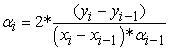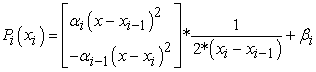3. In mathematics, a B-spline is a linear combination of positive splines with minimal compact support. The B-splines are the generalization of the Bezier curves, they can in turn be generalized by the NURBS.

4.5. The polynomials interpolators of Lagrange on the abscissa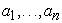are the polynomials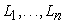defined for i=1, …, n with:We are interested in the linear combinations of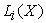. Let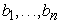be n real numbers and form the polynomial: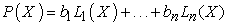6. Theorem: f a function of class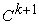on an interval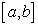and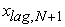the Lagrange interpolation polynomial of f at the points a=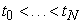=b, thenOr(t)=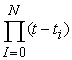7. The survival function (often called R (t)) is the complement of the cumulative distribution function (R (t) = 1-F (t)); the survival function is also sometimes referred to as a survival or reliability function (since it describes the probability of not failing or of surviving until a certain time t).

8. Any economic actor must be able to enter the market to buy or sell, but also to be free to withdraw from the market. For a new company, there must be no barriers or obstacles to its establishment or subsequently to its production activity. It must therefore be able to withdraw from the market if it deems it necessary. In economic terms, we are talking about a fluid market.

9. A process GARCH (p,q) (Generalized Autoregressive Conditionally Heteroskedastic or Conditional Heteroskedasticity) is defined as: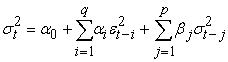Where: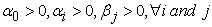(there are also conditions for stationarity: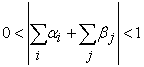)

10. (Today’s date-1)-the operation date ≤ 30

11. The acquisition date of the Treasury bill

12. (Today’s date-1)-the operation date ≤ 60

13. The FMC is an independent public authority that has civil personality and financial autonomy. The FMC is responsible for ensuring the protection of savings invested in securities.

14. 1 basis point= 10-4 (chosen arbitrarily)

15. Quantity1: the quantity of each treasury bill.

16.17. Quantity2: the sum of the treasury bills with the same code ISIN.

19. 10 and 5 basis points are chosen arbitrarily according to Tunisian bank practices.

20. The economic environment is the set of factors that affect the business of a company. We talk about "macroeconomic" factors.

a. Social factors: for example, demographics, culture, level of unemployment and patterns of consumption.

b. Political and legal factors: the legal framework governing economic activities, political decisions that affect the functioning of enterprises (for example a change in taxation).

c. Economic factors: the income of the citizens.

d. Technological factors: the frequency of technological changes likely to affect the business.

We can talk about a stable economic environment when all these factors are stable.

21. Characteristic of the person who does not want to take a risk and who will be willing to transfer it to a third party for a fee or who will refuse any asset or any action that causes him to incur a risk that he perceives as excessive due to his ability to support.

### References

  Gbongue, F., & Frederic Planchet. (2015). Analyse comparative d'une courbe des taux sans risque dans la zone CIPRES. Laboratoire de science actuarielle et financière. In article  Roncalli, T. (1998). La structure par terme des taux zéro: modélisation et implémentation numérique. Thèse Université Montesquieu Bordeaux IV. In article View Article  McENally, G.H. (1987). Estimation of coal measures rock strength using sonic and neutron logs. Geoexploration Amsterdam Volume 24 (Number 4-5), 381-395. In article View Article  Dobbie, G.M. and Wilkie, A.D. (1978) The FT-Actuaries Fixed Interest Indices. Journal of the Institute of Actuaries 105, 15-27. In article View Article  Dobbie, G.M. and Wilkie, A.D. (1979) The FT-Actuaries Fixed Interest Indices. Transactions of the Faculty of Actuaries 36, 203-213. In article View Article  Bolder D., Stréliski D. (1999). Yield Curve Modelling at the Bank of Canada. Technical Report no 84. In article View Article  McCulloch JH and LA Kochin. (1998). The Inflation Premium Implicit in the US Real and Nominal Term Structures of Interest rates. Economics Department of Ohio State University Working Paper No 98-12. In article View Article  Pansu, P. (2004). Interpolation et approximation par des B-splines. Working Paper.Université Paris-Sud. In article View Article  Carriere J. F. (1998). Withdrawal benefits under a dependent double decrement model. Astin bultin. Vol 28. No. 1, pp. 49-57. In article View Article  Nelson C.R., Siegel A.F. (1987). Parsimonious modelling of yield curves. Journal of Business, 60, 473-489. In article View Article  Düllmann, Klaus, Marliese, Uhrig-Homburg, and Marc Windfuhr. (2000). Risk structure of interest rates: an empirical analysis for deutschmark-denominated bonds. European Financial Management, Vol. 6, No. 3, 367-388. In article View Article  Pierre-E.Therond. (2013). Génération de scénarios économiques (Modélisation des taux d'intèret). Université Lyon1. In article  Vasicek, O., Fong, H. G. (1982). Term structure modeling using exponential splines. The Journal of Finance, 37 (2), 339-348. In article View Article  Brennan, M. J., and E. S. Schwartz. (1982). An Equilibrium Model of Bond Pricing and Test of Market Efficiency. Journal of Financial and Quantitative Analysis. Vol. 17, pp. 301-329. In article View Article  Ho, T. S. Y., and S.-B. Lee. (1986). Term Structure Movements and the Pricing of Interest Rate Contingent Claims. Journal of Finance, 41, pp. 1011-1029. In article View Article  Heath, David, Robert Jarrow, and Andrew Morton. (1992). Bond Pricing and the Term Structure of Interest Rates: A New Methodology for Contingent Claims Valuation. Econometrica, Vol. 60, No. 1, pp 77-105. In article View Article  Annaert, J., Anouk G.P.Claes, Mark J. K. De Ceuster, & Hairui Zhang. (2013). Estimating the spot rate curve using the Nelson-Siegel model. A ridge regression approach. Working Paper, University of Antwerp. In article View Article  Bowsher, Clive G., and Roland Meeks. (2008). The Dynamics of Economic Functions: Modelling and forecasting the yield curve. Federal Reserve Bank of Dallas, Research Department, Working Paper 0804. In article View Article  Svensson. L.E.O. (1994). Estimating and interpreting forward interest rates: Sweden 1992-1994. International monetary fund, IMF Working Paper, 1994/114. In article View Article  Racicot, F.-É., & Raymond Theoret. (2006). Les modéles HJM et LMM revisités. Cahier de recherche 08-2006. Ecole des sciences de gestion. Université du Québec à Montréal. In article View Article  Leoni, Renato. (2007). Principal component analysis. University of Florence, Department of Statistics “G.PARENTI”, Florence, 2007. In article  Wilmott, Paul. (2007). Paul Wilmott introduces quantitative finance. John Wiley and sons LTD, The Atrium, Southern Gate, Chichester, West Sussex PO19 8SQ, England. In article PubMed  Diebold, Francis X., and Canlin Li. (2006). Forecasting thr term structure of government bond yields. Journal of Econometrics 130, 337-364. In article View Article  Pennacchi, G. G. (1991). Identifying the dynamics of real interest rates and inflation: evidence using survey data. Review of financial studies, 4, 53-86. In article View Article  Babbs, S. H., and K. B. Nowman. (1997). Kalman filtering of generalized vasicek term structure models. Financial option research center, University of Warwick, Preprint 97/80. In article  Babbs, S. H., and K. B. Nowman. (1999). Kalman filtering of generalized vasicek term structure models. Journal of Financial and Quantitative Analysis. Vol. 34, NO. 1. In article View Article  Lund, J. (1997). Econometric analysis of continuous-time arbitrage-free models of the term structure of interest rates. Technical report. Department of Finance. The Aarhus School of Business. In article  Brenner R. J., R. Harjes, and K. Kroner. (1996). Another look at models of the short-term interest rate. Journal of Financial and Quantitative Analysis, 31:85-107. In article View Article  Didouni, J. (2008). Le secteur bancaire Tunisien. Tunis: HEC Carthage. IFID Master's dissertation Maghreb arabe. In article  Gilli, M., Stefan Grobe, & E. Schumann. (2010). Calibrating the Nelson-Siegel-Svensson model. Comisef Working Papers series. In article View Article  Hladikoua, H., & Jamila Radoua. (2012). Term structure modelling by using Nelson-Siegel model. European Financial and Accounting Journal. In article View Article  McCulloch, J. (1971). Measuring the term structure of interest rates. The Journal of Business. Vol. 44, issue 1, pp. 19-31. In article View Article  Mokaddem, A. (2015). Analyse sectorielle-secteur bancaire. Tunisie Valeurs. In article PubMed  Risler, J.-J. (1991). Méthodes mathématiques pour la CAO. Masson, Paris. In article PubMed  Litterman, Robert and José Scheinkman. (1991). Common Factors Affecting Bond Returns. Journal of Fixed Income, 1(1):54-61. In article View Article

Published with license by Science and Education Publishing, Copyright © 2017 Aziz Chouikh, Rania Yousfi and Chehir ChehibiThis work is licensed under a Creative Commons Attribution 4.0 International License. To view a copy of this license, visit http://creativecommons.org/licenses/by/4.0/